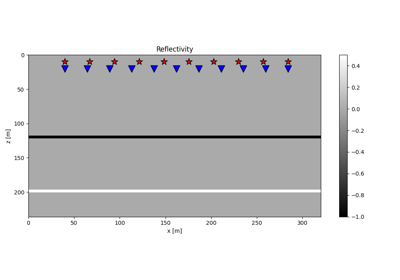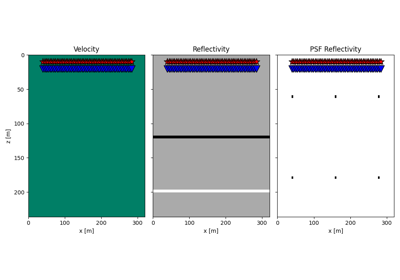# pylops.waveeqprocessing.Kirchhoff#

class pylops.waveeqprocessing.Kirchhoff(z, x, t, srcs, recs, vel, wav, wavcenter, y=None, mode='eikonal', wavfilter=False, dynamic=False, trav=None, amp=None, aperture=None, angleaperture=90.0, anglerefl=None, snell=None, engine='numpy', dtype='float64', name='K')[source]#

Kirchhoff Demigration operator.

Kirchhoff-based demigration/migration operator. Uses a high-frequency approximation of Green’s function propagators based on trav.

Parameters
znumpy.ndarray

Depth axis

xnumpy.ndarray

Spatial axis

tnumpy.ndarray

Time axis for data

srcsnumpy.ndarray

Sources in array of size $$\lbrack 2 (3) \times n_s \rbrack$$ The first axis should be ordered as (y,) x, z.

recsnumpy.ndarray

Receivers in array of size $$\lbrack 2 (3) \times n_r \rbrack$$ The first axis should be ordered as (y,) x, z.

vel

Velocity model of size $$\lbrack (n_y\,\times)\; n_x \times n_z \rbrack$$ (or constant)

wavnumpy.ndarray

Wavelet.

wavcenterint

Index of wavelet center

ynumpy.ndarray

Additional spatial axis (for 3-dimensional problems)

modestr, optional

Computation mode (analytic, eikonal or byot, see Notes for more details)

wavfilterbool, optional

New in version 2.0.0.

Apply wavelet filter (True) or not (False)

dynamicbool, optional

New in version 2.0.0.

Include dynamic weights in computations (True) or not (False). Note that when mode=byot, the user is required to provide such weights in amp.

travnumpy.ndarray or tuple, optional

Traveltime table of size $$\lbrack (n_y) n_x n_z \times n_s n_r \rbrack$$ or pair of traveltime tables of size $$\lbrack (n_y) n_x n_z \times n_s \rbrack$$ and $$\lbrack (n_y) n_x n_z \times n_r \rbrack$$ (to be provided if mode='byot'). Note that the latter approach is recommended as less memory demanding than the former.

ampnumpy.ndarray, optional

New in version 2.0.0.

Amplitude table of size $$\lbrack (n_y) n_x n_z \times n_s n_r \rbrack$$ or pair of amplitude tables of size $$\lbrack (n_y) n_x n_z \times n_s \rbrack$$ and $$\lbrack (n_y) n_x n_z \times n_r \rbrack$$ (to be provided if mode='byot'). Note that the latter approach is recommended as less memory demanding than the former.

aperturefloat or tuple, optional

New in version 2.0.0.

Maximum allowed aperture expressed as the ratio of offset over depth. If None, no aperture limitations are introduced. If scalar, a taper from 80% to 100% of aperture is applied. If tuple, apertures below the first value are accepted and those after the second value are rejected. A tapering is implemented for those between such values.

angleaperturefloat or tuple, optional

New in version 2.0.0.

Maximum allowed angle (either source or receiver side) in degrees. If None, angle aperture limitations are not introduced. See aperture for implementation details regarding scalar and tuple cases.

anglereflnp.ndarray, optional

New in version 2.0.0.

Angle between the normal of the reflectors and the vertical axis in degrees

snellfloat or tuple, optional

New in version 2.0.0.

Threshold on Snell’s law evaluation. If larger, the source-receiver-image point is discarded. If None, no check on the validity of the Snell’s law is performed. See aperture for implementation details regarding scalar and tuple cases.

enginestr, optional

Engine used for computations (numpy or numba).

dtypestr, optional

Type of elements in input array.

namestr, optional

New in version 2.0.0.

Name of operator (to be used by pylops.utils.describe.describe)

Raises
NotImplementedError

If mode is neither analytic, eikonal, or byot

Notes

The Kirchhoff demigration operator synthesizes seismic data given a propagation velocity model $$v$$ and a reflectivity model $$m$$. In forward mode , :

$d(\mathbf{x_r}, \mathbf{x_s}, t) = \widetilde{w}(t) * \int_V G(\mathbf{x_r}, \mathbf{x}, t) m(\mathbf{x}) G(\mathbf{x}, \mathbf{x_s}, t)\,\mathrm{d}\mathbf{x}$

where $$m(\mathbf{x})$$ represents the reflectivity at every location in the subsurface, $$G(\mathbf{x}, \mathbf{x_s}, t)$$ and $$G(\mathbf{x_r}, \mathbf{x}, t)$$ are the Green’s functions from source-to-subsurface-to-receiver and finally $$\widetilde{w}(t)$$ is a filtered version of the wavelet $$w(t)$$  (or the wavelet itself when wavfilter=False). In our implementation, the following high-frequency approximation of the Green’s functions is adopted:

$G(\mathbf{x_r}, \mathbf{x}, \omega) = a(\mathbf{x_r}, \mathbf{x}) e^{j \omega t(\mathbf{x_r}, \mathbf{x})}$

where $$a(\mathbf{x_r}, \mathbf{x})$$ is the amplitude and $$t(\mathbf{x_r}, \mathbf{x})$$ is the traveltime. When dynamic=False the amplitude is disregarded leading to a kinematic-only Kirchhoff operator.

$d(\mathbf{x_r}, \mathbf{x_s}, t) = \tilde{w}(t) * \int_V e^{j \omega (t(\mathbf{x_r}, \mathbf{x}) + t(\mathbf{x}, \mathbf{x_s}))} m(\mathbf{x}) \,\mathrm{d}\mathbf{x}$

On the other hand, when dynamic=True, the amplitude scaling is defined as $$a(\mathbf{x}, \mathbf{y})=\frac{1}{\|\mathbf{x} - \mathbf{y}\|}$$, that is, the reciprocal of the distance between the two points, approximating the geometrical spreading of the wavefront. Moreover an angle scaling is included in the modelling operator added as follows:

$d(\mathbf{x_r}, \mathbf{x_s}, t) = \tilde{w}(t) * \int_V a(\mathbf{x}, \mathbf{x_s}) a(\mathbf{x}, \mathbf{x_r}) \frac{|cos \theta_s + cos \theta_r|} {v(\mathbf{x})} e^{j \omega (t(\mathbf{x_r}, \mathbf{x}) + t(\mathbf{x}, \mathbf{x_s}))} m(\mathbf{x}) \,\mathrm{d}\mathbf{x}$

where $$\theta_s$$ and $$\theta_r$$ are the angles between the source-side and receiver-side rays and the normal to the reflector at the image point (or the vertical axis at the image point when reflslope=None), respectively.

Depending on the choice of mode the traveltime and amplitude of the Green’s function will be also computed differently:

• mode=analytic or mode=eikonal: traveltimes, geometrical spreading, and angles are computed for every source-image point-receiver triplets and the Green’s functions are implemented from traveltime look-up tables, placing scaled reflectivity values at corresponding source-to-receiver time in the data.

• byot: bring your own tables. Traveltime table are provided directly by user using trav input parameter. Similarly, in this case one can provide their own amplitude scaling amp (which should include the angle scaling too).

Three aperture limitations have been also implemented as defined by:

• aperture: the maximum allowed aperture is expressed as the ratio of offset over depth. This aperture limitation avoid including grazing angles whose contributions can introduce aliasing effects. A taper is added at the edges of the aperture;

• angleaperture: the maximum allowed angle aperture is expressed as the difference between the incident or emerging angle at every image point and the vertical axis (or the normal to the reflector if anglerefl is provided. This aperture limitation also avoid including grazing angles whose contributions can introduce aliasing effects. Note that for a homogenous medium and slowly varying heterogenous medium the offset and angle aperture limits may work in the same way;

• snell: the maximum allowed snell’s angle is expressed as the absolute value of the sum between incident and emerging angles defined as in the angleaperture case. This aperture limitation is introduced to turn a scattering-based Kirchhoff engine into a reflection-based Kirchhoff engine where each image point is not considered as scatter but as a local horizontal (or straight) reflector.

Finally, the adjoint of the demigration operator is a migration operator which projects data in the model domain creating an image of the subsurface reflectivity.

1

Bleistein, N., Cohen, J.K., and Stockwell, J.W.. “Mathematics of Multidimensional Seismic Imaging, Migration and Inversion”, 2000.

2

Santos, L.T., Schleicher, J., Tygel, M., and Hubral, P. “Seismic modeling by demigration”, Geophysics, 65(4), pp. 1281-1289, 2000.

3

Safron, L. “Multicomponent least-squares Kirchhoff depth migration”, MSc Thesis, 2018.

Attributes
shapetuple

Operator shape

explicitbool

Operator contains a matrix that can be solved explicitly (True) or not (False)

Methods

 __init__(z, x, t, srcs, recs, vel, wav, ...) adjoint() apply_columns(cols) Apply subset of columns of operator cond([uselobpcg]) Condition number of linear operator. conj() Complex conjugate operator div(y[, niter, densesolver]) Solve the linear problem $$\mathbf{y}=\mathbf{A}\mathbf{x}$$. dot(x) Matrix-matrix or matrix-vector multiplication. eigs([neigs, symmetric, niter, uselobpcg]) Most significant eigenvalues of linear operator. matmat(X) Matrix-matrix multiplication. matvec(x) Matrix-vector multiplication. reset_count() Reset counters rmatmat(X) Matrix-matrix multiplication. rmatvec(x) Adjoint matrix-vector multiplication. todense([backend]) Return dense matrix. toimag([forw, adj]) Imag operator toreal([forw, adj]) Real operator tosparse() Return sparse matrix. trace([neval, method, backend]) Trace of linear operator. transpose()

## Examples using pylops.waveeqprocessing.Kirchhoff#15. Least-squares migration

15. Least-squares migration20. Image Domain Least-squares migration

20. Image Domain Least-squares migration